# ML Aggarwal Class 8 Solutions for ICSE Maths Chapter 19 Data Handling Ex 19.1

## ML Aggarwal Class 8 Solutions for ICSE Maths Chapter 19 Data Handling Ex 19.1

Question 1.
The result of a survey of 200 people about their favourite fruit is given below: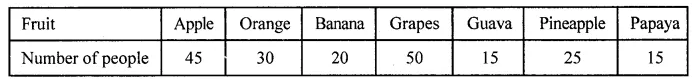Represent the above data by a bar graph.
Solution: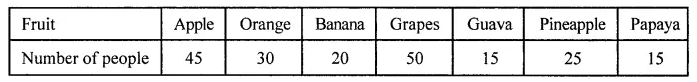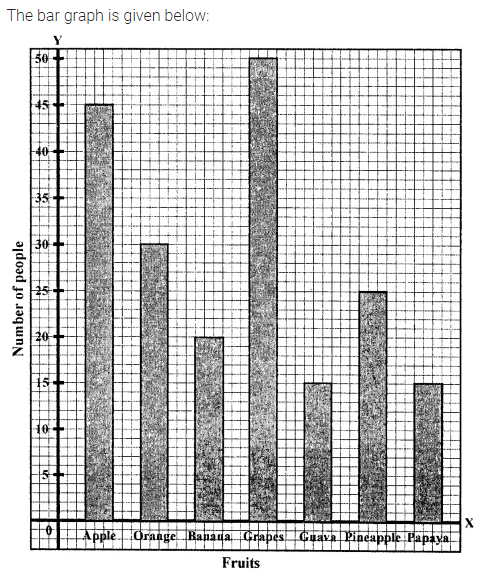Question 2.
Mr Khurana has two kitchen appliance stores. He compares the sales of two stores during a month and recovered as given below:Represent the above data by a double bar graph.
Solution: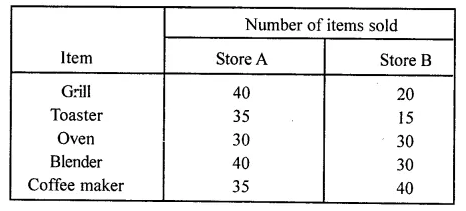Question 3.
The number of goals scored by a football team in different matches is given below:
3, 1, 0, 4, 6, 0, 0, 1, 1, 2, 2, 3, 5, 1, 2, 0, 1, 0, 2, 3, 9, 2, 0, 1, 0, 1, 4, 1, 0, 2, 5, 1, 2, 2, 3, 1, 0, 0, 0, 1, 1, 0, 2, 3, 0, 1, 5, 2, 0
Make a frequency distribution table using tally marks.
Solution: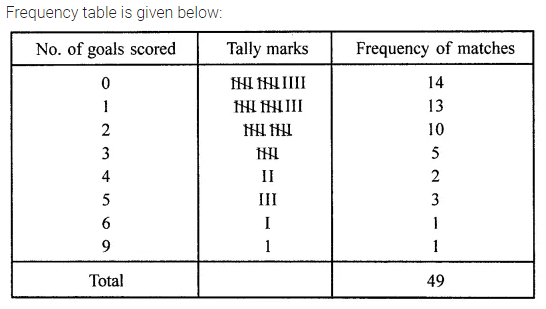Question 4.
Given below a bar graph:(i) What is the information given by the bar graph?
(ii) On which item the expenditure is maximum?
(iii) On which item the expenditure is minimum?
(iv) State whether true or false:
Expenditure on education is twice the expenditure on clothing.
Solution: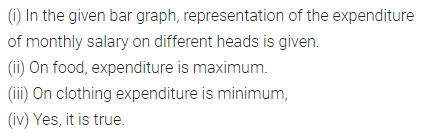Question 5.
Given below a double bar graph.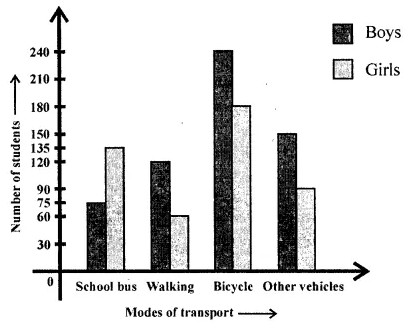(i) What is the information given by the double graph?
(ii) Which mode of transport girls using more than the boys?
(iii) Which mode of transport boys using the most?
(iv) In which mode of transport number of girls is half the number of boys?
Solution: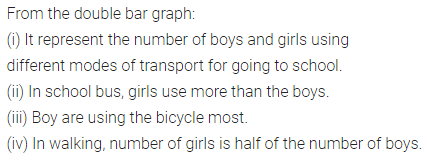Question 6.
Using class intervals 0-5, 5-10, construct the frequency distribution table for the following
data:
13, 6, 12, 9, 11, 14, 2, 8, 18, 16, 9, 13, 17, 11, 19, 6, 7, 12, 22, 21, 18, 1, 8, 12, 18.
Solution:Question 7.
Given below are the marks secured by 35 students in a surprise test:
41, 32, 35, 21, 11, 47, 42, 00, 05, 18, 25, 24, 29, 38, 30, 04, 14, 24, 34, 44, 48, 33, 36, 38, 41, 48, 08, 34, 39, 11, 13, 27, 26, 43, 03.
Taking class intervals 0-10, 10-20, …….. construct frequency distribution table. Find the number
of students obtaining below 20 marks.
Solution: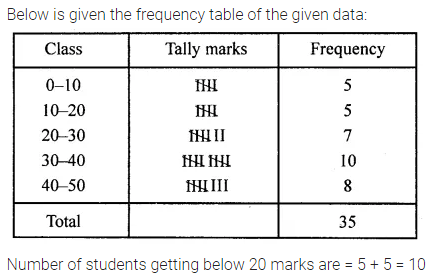Question 8.
The electricity bills (in ?) of 40 houses in a locality are given below:
78, 87,81,52, 59, 65, 101, 108, 115, 95, 98, 65,62, 121, 128, 63,76, 84, 89,91,65, 101,95,81, 87, 105, 129, 92, 75, 105, 78, 72, 107, 116, 127, 100, 80, 82, 61, 118 Construct a grouped frequency distribution table of class size 10.
Class intervals (Electricity bill in ?) Tally marks Frequency (Number of houses)
Solution:Question 9.
Draw a histogram for the frequency table made for data in Question 8, and answer the following questions:
(i) Which group has the maximum number of houses?
(ii) How many houses pay less than ₹ 100?
(iii) How many houses pay ₹ 100 or more?
Solution: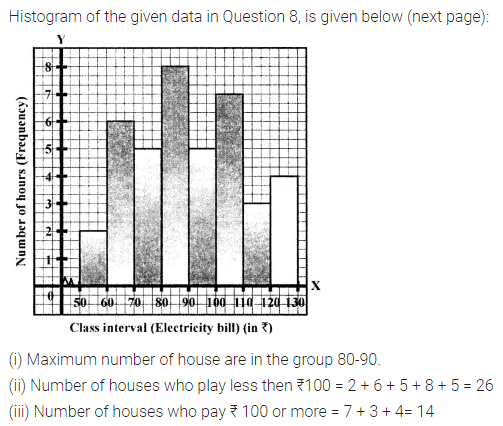Question 10.
The weights of 29 patients in a hospital were recorded as follows:Draw a histogram to represent this data visually.
Solution: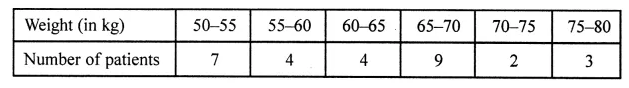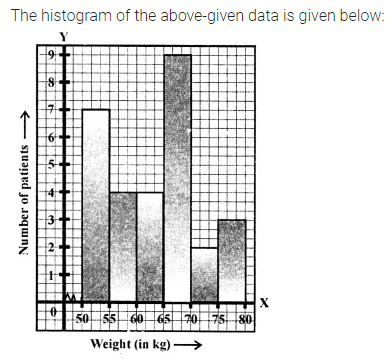Question 11.
In a study of diabetic patients, the following data was obtained:Represent the above data by a histogram.
Solution: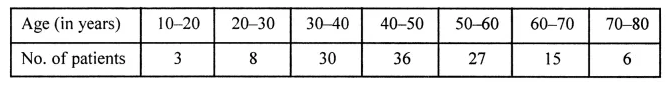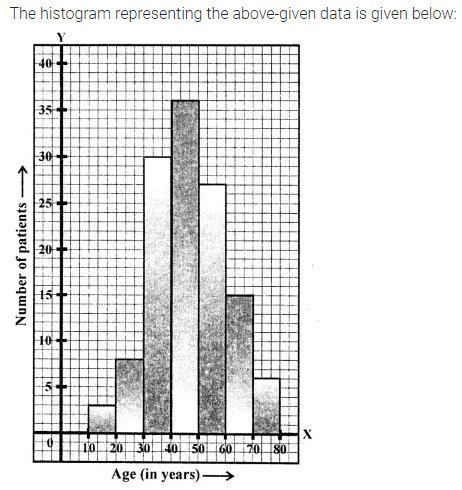Question 12.
The histogram showing the weekly wages (in ₹) of workers in a factory is given alongside: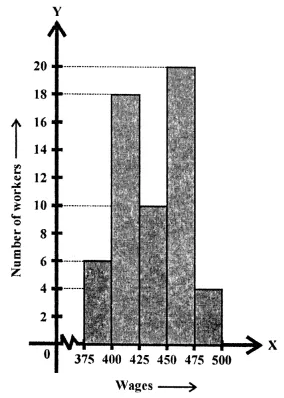(i) What is the frequency of class 400-425?
(ii) What is the class having a minimum frequency?
(iii) How many workers get more than ₹425?
(iv) How many workers get less than ₹475?
(v) Number of workers whose weekly wages are more than or equal to ₹400 but less than ₹450.
Solution:Question 13.
The number of hours for which students of a particular class watched television during holidays is shown in the histogram below.(i) For how many hours did the maximum number of students watch T.V.?
(ii) How many students watched T.V. for less than 4 hours?
(iii) How many students spent more than 5 hours in watching T.V.?
(iv) How many students spent more than 2 hours but less than 4 hours in watching T.V.?
Solution: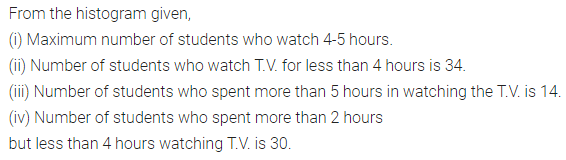Question 14.
The number of literate females in the age group of 10 to 40 years in a town is shown in the histogram alongside.